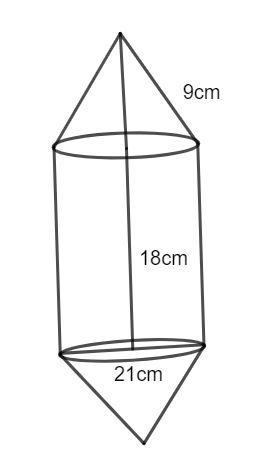QUESTION

# A petrol tank is a cylinder of base diameter 21 cm and length 18 cm fitted with conical ends each of axis length 9 cm. Determine the capacity of the tank.

Hint: The formula for the volume of the cylinder is $\pi {r^2}h$ and the volume of the cone is $\dfrac{1}{3}\pi {r^2}h$. Using these formulae calculate the volume of the entire tank.The volume of a cylinder of height ‘h’ and radius ‘r’ is given as follows:

$V = \pi {r^2}h............(1)$

The diameter of the base is given to be 21 cm. The radius is half of the diameter. Hence, we have:

$r = \dfrac{{21}}{2}$

$r = 10.5cm............(2)$

The volume of the cylinder with radius 10.5 cm and length 18 cm is given using equation (1) as follows:

${V_1} = \dfrac{{22}}{7}{(10.5)^2}(18)$

Simplifying, we get:

${V_1} = 6237c{m^3}..........(3)$

The volume of a cone with radius r and height h is given as follows:

$V = \dfrac{1}{3}\pi {r^2}h.........(4)$

The volume of two cones of height 9 cm and radius 10.5 cm is given from equation (4) as follows:

${V_2} = 2 \times \dfrac{1}{3}.\dfrac{{22}}{7}{(10.5)^2}(9)$

Simplifying, we get:

${V_2} = 2079c{m^3}............(5)$

The total volume of the tank is given from equation (3) and equation (5) as follows:

$V = {V_1} + {V_2}$

$V = 6237 + 2097$

$V = 8316c{m^3}$

Hence, the volume of the petrol tank is 8316 $c{m^3}$.

Note: There are two cones fitted at two ends, you may forget to calculate the volume for both the cones. The height of the cones and the cylinders are different.
It is given that a petrol tank is a cylinder fitted with conical ends. Hence, the tank can be divided into a cylinder with two cones. The volume of the petrol tank is the sum of the volumes of the cylinder and the two cones.